### Active-Set Method

Active-set methods differ from interior point methods in that no barrier term is used to ensure that the algorithm remains interior with respect to the inequality constraints. Instead, attempts are made to learn the true active set. For simplicity, use the same initial slack formulation used by the interior point method description,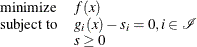where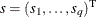is the vector of slack variables, which are required to be nonnegative. Begin by absorbing the equality constraints as before into a penalty function, but keep the slack bound constraints explicitly: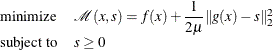whereis a positive parameter. Given a solution pair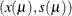for the preceding problem, you can define the active-set projection matrix P as follows: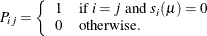Thenis also a solution of the equality constraint subproblem: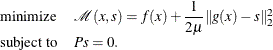The minimizer of the preceding subproblem must be a stationary point of the Lagrangian function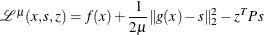which gives the optimality equations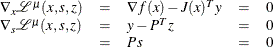where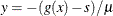. Using the second equation, you can simplify the preceding equations to get the following optimality conditions for the bound-constrained penalty subproblem: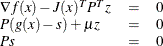Using the third equation directly, you can reduce the system further to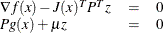At iteration k, the primal-dual active-set algorithm approximately solves the preceding system by using Newton’s method. The Newton system is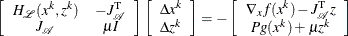where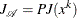and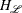denotes the Hessian of the Lagrangian function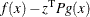. The solution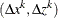of the Newton system provides a direction to move from the current iteration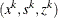to the next,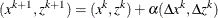where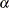is the step length along the Newton direction. The corresponding slack variable update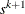is defined as the solution to the following subproblem whose solution can be computed analytically: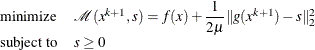The step lengthis then determined in a similar manner to the preceding interior point approach. At each iteration, the definition of the active-set projection matrix P is updated with respect to the new value of the constraint function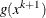. For large-scale NLP, the computational bottleneck typically arises in seeking to solve the Newton system. Thus active-set methods can achieve substantial computational savings when the size of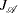is much smaller than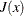; however, convergence can be slow if the active-set estimate changes combinatorially. Further, the active-set algorithm is often the superior algorithm when only bound constraints are present. In practice, both the interior point and active-set approach incorporate more sophisticated merit functions than those described in the preceding sections; however, their description is beyond the scope of this document. See Gill and Robinson (2010) for further reading.